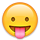13»

XRinger wrote: »
It must be a heat pump, because 624 watts is 2129.176 BTUh,
2,662 BTUh is equal to 780.1552 watts.Interesting... it's rated .78KVa - I used an online converter to convert that to watts.

kVA is not kWatts... More or less. They are two different measurements for different uses.

The "real" equation for power is:
• Watts = Volts * Amps (DC)
• Watts = Volts * Amps * Cosine of the angle between Volts and Amps (current)
• Watts = Volts * Amps * Power Factor
Power Factor can equal Cosine of the V/A angle for linear circuits (motors, inductors, capacitive circuits, etc.).

Or Power Factor is a measured/mathematical term used to describe the V/A relationship for non-linear circuits (typically computer power supplies, lamp dimmers, battery chargers, etc.).

Looking at the picture I posted earlier:You see that the blue line (current) is not a sine wave at all...

Why is this important--Volts*Amps (or amps directly) better describes the current carrying capacity of wiring, how generator windings/magnetics behave, waste heat from I^2*R heating, etc. can be modeled/defined/designed...

Watts or Power is the amount of energy the system is using...

So, it is very possible to have a circuit that pulls 1 amps at 120 VAC, but with a Power Factor of 0.6 (motor, CFL lamp, etc.) and you get:
• 1 amp * 120 VAC = 120 VA (wiring design, generator/inverter maximum VA rating, etc.).
• 1 amp * 120 VAC * 0.6 PF = 72 Watts (amount of heat/energy the load is taking, how much fuel the genset will use, how much energy an inverter will pull from the battery bank).
-Bill
Near San Francisco California: 3.5kWatt Grid Tied Solar power system+small backup genset
• Solar Expert Posts: 10,300 ✭✭✭✭
XRinger wrote: »
It must be a heat pump, because 624 watts is 2129.176 BTUh,
2,662 BTUh is equal to 780.1552 watts.taint quite right as a watt is about 3.4btu.